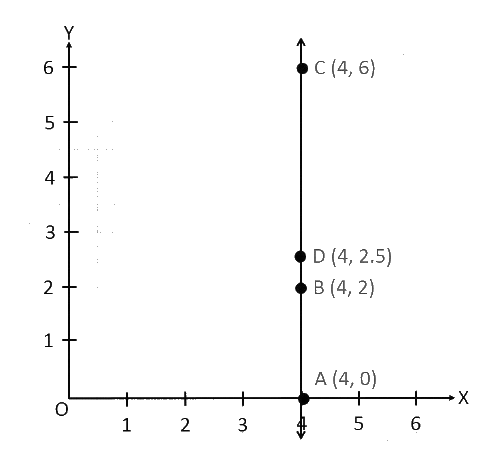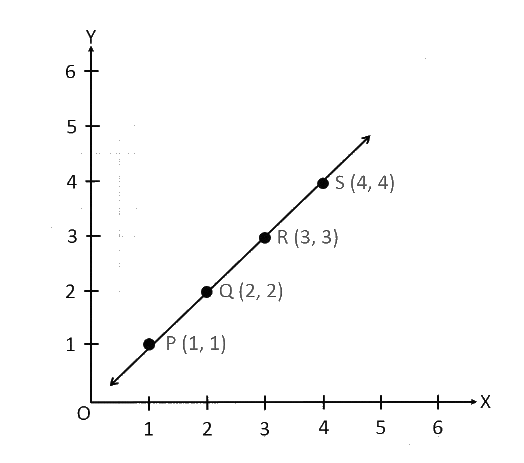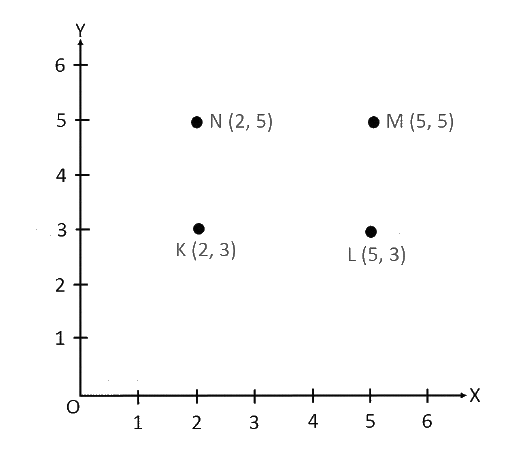# Ex.15.2 Q1 Introduction to Graphs Solutions - NCERT Maths Class 8

Go back to  'Ex.15.2'

## Question

Plot the following points on a graph sheet. Verify if they lie on a line

(a)

$$\rm{A(4,0),{{B}}(4,2),{{C}}(4,6),{{D}}(4,2.5)}$$

(b)

$$\rm{P(1,1),\;{{Q}}(2,2),\;{{R}}(3,3),\;{{S}}(4,4)}$$

(c)

$$\rm{K(2,3),\; L( (5,3),\;M(5,5),\;N(2,5)}$$

## Text Solution

Reasoning:

Consider $$x$$-axis and $$y$$-axis as a number line starting from ($$0,0$$). And locate the given points.

Steps:

(a) We can plot the given points and join the consecutive points on a graph paper as follows.From the graph, it can be observed that the points $$A, B, C,$$ and $$D$$ lie on the same line.

(b) We can plot the given points and join the consecutive points on a graph paper as follows.Hence, points $$P, Q, R,$$ and $$S$$ lie on the same line.

(c) We can plot the given points and join the consecutive points on a graph paper as follows.Hence, points$$K, L, M,$$and $$N$$ are not lying on the same line.

Learn from the best math teachers and top your exams

• Live one on one classroom and doubt clearing
• Practice worksheets in and after class for conceptual clarity
• Personalized curriculum to keep up with school